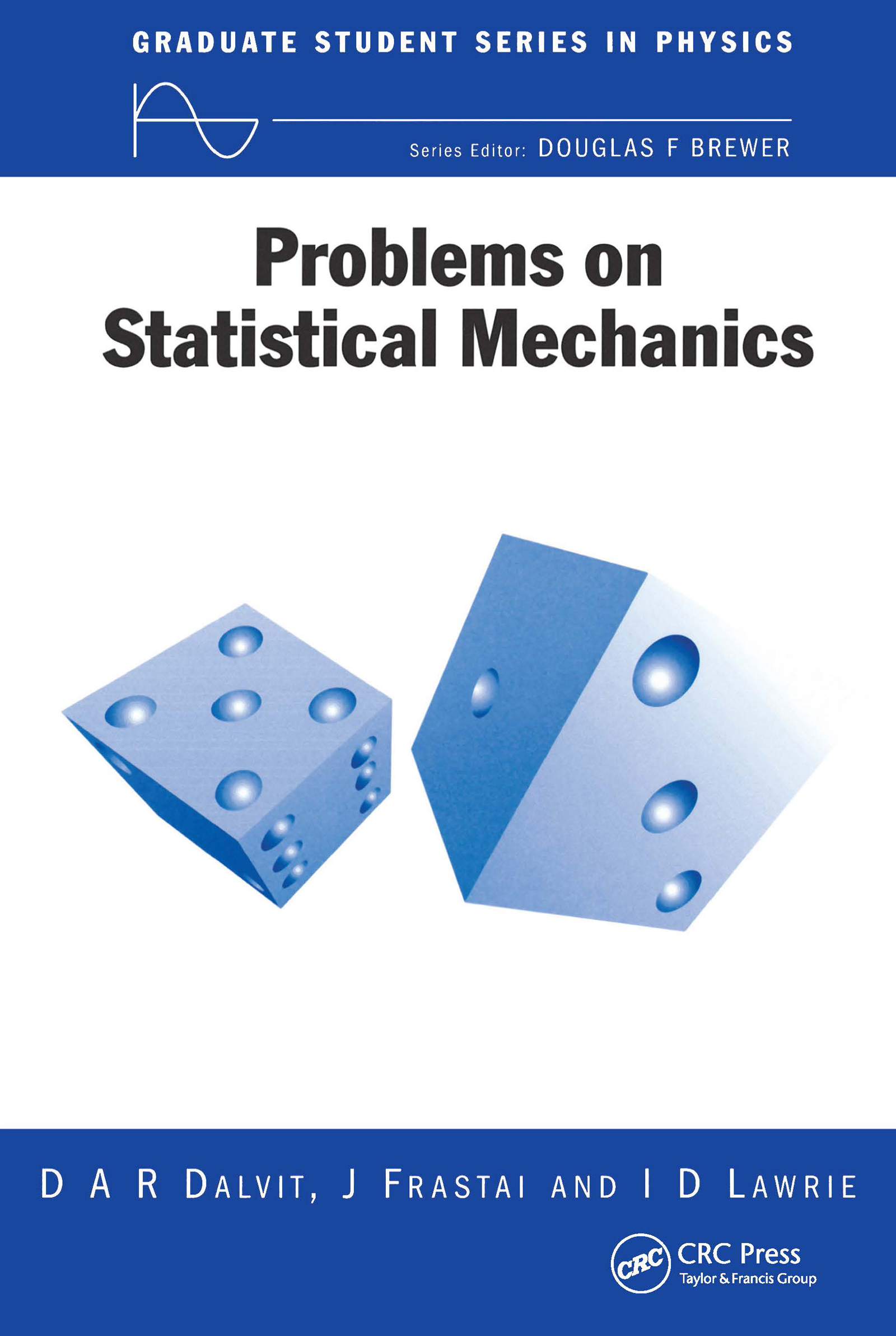Problems on Statistical Mechanics

1st Edition

CRC Press

283 pages

Purchasing Options:\$ = USD
Paperback: 9780750305211
pub: 1999-01-01
SAVE ~\$17.39
\$86.95
\$69.56
x

FREE Standard Shipping!

Description

A thorough understanding of statistical mechanics depends strongly on the insights and manipulative skills that are acquired through the solving of problems. Problems on Statistical Mechanics provides over 120 problems with model solutions, illustrating both basic principles and applications that range from solid-state physics to cosmology. An introductory chapter provides a summary of the basic concepts and results that are needed to tackle the problems, and also serves to establish the notation that is used throughout the book. The problems themselves occupy five chapters, progressing from the simpler aspects of thermodynamics and equilibrium statistical ensembles to the more challenging ideas associated with strongly interacting systems and nonequilibrium processes. Comprehensive solutions to all of the problems are designed to illustrate efficient and elegant problem-solving techniques. Where appropriate, the authors incorporate extended discussions of the points of principle that arise in the course of the solutions. The appendix provides useful mathematical formulae.

Reviews

"The best way to learn a skill is by active practice. This wonderful collection of statistical mechanics problems, compiled by the trio, supplies a host of interesting questions and carefully worked-out solutions. Anyone with a basic background in statistical mechanics and thermodynamics can pick up the book and start practice sessions."

- Henrik Jeldtoft Jensen, Mathematics, Physics and Engineering

Preliminaries

Review of theoretical definitions and formulae

Notation used throughout the book

Thermodynamics

First and second law

Thermodynamic potentials and quantities

Maxwell relations

Simple thermodynamic processes

Statistical Ensembles

Microcanonical, canonical, and grand canonical ensembles

Connection with thermodynamics

Equipartition theorem

Noninteracting gases of classical particles

Noninteracting lattice-type systems

Gases of molecules

Quantum Statistics

Statistics of indistinguishable particles, bosons, and fermions

Density of states

Debye's models for solids

Bose condensation

Fermi gas

Interacting Systems

Classical gases and virial coefficients

Critical exponents in phase transitions

Ising type models and Heisenberg modes

Exact and mean field approaches

Nonequilibrium Systems

Random walk

Markov chains

Master equation

Diffusion phenomena

Boltzmann transport equation

Relaxation time approximation

Appendix: Useful Mathematical Formulae

Index